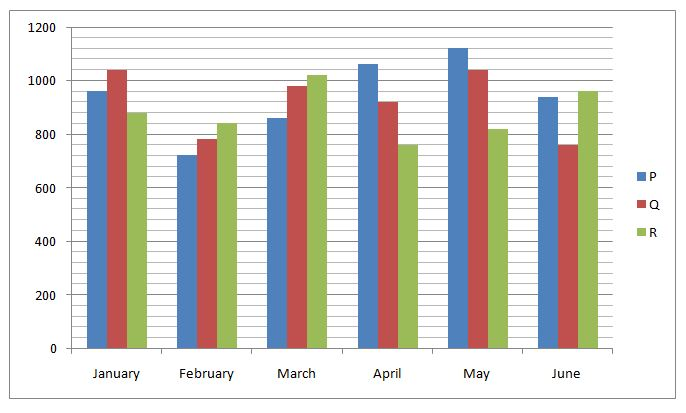# IBPS RRB Clerk/EPFO Assistant/LIC ADO Prelims Quantitative Aptitude Questions 2019 (Day-03)

Dear Aspirants, Our IBPS Guide team is providing new series of Quantitative Aptitude Questions for IBPS RRB Clerk/EPFO Assistant/LIC ADO Prelims 2019 so the aspirants can practice it on a daily basis. These questions are framed by our skilled experts after understanding your needs thoroughly. Aspirants can practice these new series questions daily to familiarize with the exact exam pattern and make your preparation effective.

##### LIC ADO Prelims Free Mock Test

[WpProQuiz 6577]

Directions (Q. 1 – 5): What value should come in place of (?) in the following number series?

1) 73, 37, 38, 58.5, 119, ?

a) 300

b) 324

c) 348

d) 272

e) 290

2) 18, 32, 105, 404, 2045, ?

a) 10586

b) 14752

c) 8625

d) 12234

e) 13865

3) 24, 36, 54, 81, 121.5, ?

a) 196

b) 218

c) 232

d) 174.75

e) 182.25

4) 1386, 1386, 693, 231, 57.75, ?

a) 18.25

b) 11.55

c) 24.65

d) 15.45

e) 27.35

5) 264, 1595, 2324, 2667, 2792, ?

a) 3157

b) 3392

c) 2819

d) 2675

e) 3068

Directions (Q. 6 – 10): Study the following information carefully and answer the given questions.

The following bar graph shows the total distance (In Km) travelled by 3 different persons in 5 different months.6) Find the ratio between the total distance travelled by P in the month of January, March and May together to that of total distance travelled by R in the month of February, April and June together?

a) 143: 144

b) 147: 128

c) 141: 128

d) 128: 141

e) None of these

7) Find the difference between the total distance travelled by Q in the month of January and February together to that of total distance travelled by the same person in the month of May and June together?

a) 60 km

b) 40 km

c) 80 km

d) 20 km

e) None of these

8) Total distance travelled by all the 3 persons in the month of February is approximately what percentage more/less than the total distance travelled by all the 3 persons in the month of April?

a) 25 % more

b) 25 % less

c) 15 % less

d) 15 % more

e) 40 % more

9) Find the average distance travelled by person Q in all the given months together?

a) 920 km

b) 1050 km

c) 1180 km

d) 1370 km

e) None of these

10) Total distance travelled by person P and Q together in the month of March is approximately what percentage of total distance travelled by person Q and R together in the month of May?

a) 85 %

b) 70 %

c) 120 %

d) 135 %

e) 100 %

Directions (1-5):

73 * 0.5 + 0.5 = 37

37 * 1 + 1 = 38

38 * 1.5 + 1.5 = 58.5

58.5 * 2 + 2 = 119

119 * 2.5 + 2.5 = 300

18 * 2 – 22 = 32

32 * 3 + 32 = 105

105 * 4 – 42 = 404

404 * 5 + 52 = 2045

2045 * 6 – 62 = 12234

24 * 1.5 = 36

36 * 1.5 = 54

54 * 1.5 = 81

81 * 1.5 = 121.5

121.5 * 1.5 = 182.25

1386 ÷ 1 = 1386

1386 ÷ 2 = 693

693 ÷ 3 = 231

231 ÷ 4 = 57.75

57.75 ÷ 5 = 11.55

264 + 1331 (113) = 1595

1595 + 729 (93) = 2324

2324 + 343 (73) = 2667

2667 + 125 (53) = 2792

2792 + 27 (33) = 2819

Directions (6-10):

The total distance travelled by P in the month of January, March and May together

= > 960 + 860 + 1120 = 2940 km

The total distance travelled by R in the month of February, April and June together

= > 840 + 760 + 960 = 2560 km

Required ratio = 2940 : 2560 = 147: 128

The total distance travelled by Q in the month of January and February together

= > 1040 + 780 = 1820 km

The total distance travelled by the same person in the month of May and June together

= > 1040 + 760 = 1800 km

Required difference = 1820 – 1800 = 20 km

Total distance travelled by all the 3 persons in the month of February

= > 720 + 780 + 840 = 2340 km

Total distance travelled by all the 3 persons in the month of April

= > 1060 + 920 + 760 = 2740 km

Required % = [(2740 – 2340) / 2740] * 100 = 15 % less

The average distance travelled by person Q in all the given months together

= > (1040 + 780 + 980 + 920 + 1040 + 760) / 6

= > 5520/6 = 920 km

The total distance travelled by person P and Q together in the month of March

= > 860 + 980 = 1840 km

The total distance travelled by person Q and R together in the month of May

= > 1040 + 820 = 1860 km

Required % = (1840 / 1860) * 100 = 98.92 % = 100 %